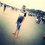# Need Help(IIT Problem)

Q - If $$f(x) = \displaystyle\int_1^x \dfrac{\ln t}{1 + t} \text{dt}$$ where $$x > 0$$ ,
Then the value of x satisfying the equation $f(x) + f\left(\dfrac{1}{x}\right) = 2$ is

I attacked the question in this way,
$f(x) + f\left(\dfrac{1}{x}\right) = 2$
Differentiating both sides with respect to x.
$f'(x) + f'\left(\dfrac{1}{x}\right)\cdot \dfrac{-1}{x^2} = 0 \quad \quad ...1$

Applying leibniz theorem in the given integral,
$f'(x) = \dfrac{\ln x}{1+x} \quad \quad f'\left(\dfrac{1}{x}\right) = \cfrac{\ln\left(\frac{1}{x}\right)}{1 + \frac{1}{x}} = \dfrac{-x \ln x}{1 + x}$
Substituting values of $f(x) \ and \ f'\left(\dfrac{1}{x}\right)$ in equation 1 ,

$\dfrac{\ln x}{1+x} = \dfrac{x \ln x}{1 + x}$
$\boxed{x = 1}$Note by Akhil Bansal
5 years, 10 months ago

This discussion board is a place to discuss our Daily Challenges and the math and science related to those challenges. Explanations are more than just a solution — they should explain the steps and thinking strategies that you used to obtain the solution. Comments should further the discussion of math and science.

When posting on Brilliant:

• Use the emojis to react to an explanation, whether you're congratulating a job well done , or just really confused .
• Ask specific questions about the challenge or the steps in somebody's explanation. Well-posed questions can add a lot to the discussion, but posting "I don't understand!" doesn't help anyone.
• Try to contribute something new to the discussion, whether it is an extension, generalization or other idea related to the challenge.

MarkdownAppears as
*italics* or _italics_ italics
**bold** or __bold__ bold
- bulleted- list
• bulleted
• list
1. numbered2. list
1. numbered
2. list
Note: you must add a full line of space before and after lists for them to show up correctly
paragraph 1paragraph 2

paragraph 1

paragraph 2

[example link](https://brilliant.org)example link
> This is a quote
This is a quote
    # I indented these lines
# 4 spaces, and now they show
# up as a code block.

print "hello world"
# I indented these lines
# 4 spaces, and now they show
# up as a code block.

print "hello world"
MathAppears as
Remember to wrap math in $$ ... $$ or $ ... $ to ensure proper formatting.
2 \times 3 $2 \times 3$
2^{34} $2^{34}$
a_{i-1} $a_{i-1}$
\frac{2}{3} $\frac{2}{3}$
\sqrt{2} $\sqrt{2}$
\sum_{i=1}^3 $\sum_{i=1}^3$
\sin \theta $\sin \theta$
\boxed{123} $\boxed{123}$

Sort by:

The error in your method is that you differentiated both sides of a non-identical equation. Only functions (implicit or explicit) and identities can be differentiated (this is one of the basic rules of differentiation).

The statement $f(x)+f(1/x)=2$ is an equation in terms of $x$ which holds true for a finite set of values of $x$, i.e., the equality here is non-identical. As such, you can't differentiate it w.r.t $x$.

The answers on this post highlights the fallacy of differentiating an equation which is not an identity.

- 5 years, 10 months ago

Thanks both of you.

- 5 years, 10 months ago

I think that you first need to look at the function $g(x) = f(x) + f\left(\dfrac{1}{x}\right)$ in general.

Then $g'(x) = f'(x) + f'\left(\dfrac{1}{x}\right)*\dfrac{-1}{x^{2}} = \dfrac{\ln(x)}{1 + x} + \dfrac{\ln(x)}{x(1 + x)} = \dfrac{x\ln{x} + \ln(x)}{x(1 + x)} = \dfrac{\ln(x)}{x}.$

Integrate using the substitution $u = \ln(x)$ to find that $g(x) = \dfrac{1}{2}(\ln(x))^{2} + C.$

Next, since $g(1) = 0$ we see that $C = 0,$ and so $g(x) = \dfrac{1}{2}(\ln(x))^{2}.$

Finally, for this to equal $2$ we need to have $(\ln(x))^{2} = 4 \Longrightarrow \ln(x) = \pm 2,$

and so either $x = e^{2}$ or $x = \dfrac{1}{e^{2}}.$

- 5 years, 10 months ago

I really liked your solution,great observation that $g(1) = 0$ .Thanks!

- 5 years, 10 months ago

- 5 years, 10 months ago

I am getting $x=e^2$

- 5 years, 10 months ago

Correct answer is $e^2$ and $e^{-2}$

- 5 years, 10 months ago

akhil i am connected brillant now

- 5 years, 9 months ago

Good,sabse pehle mje apna phone number de..

- 5 years, 9 months ago

are you subscribed to brilliant

- 5 years, 9 months ago

No

- 5 years, 9 months ago